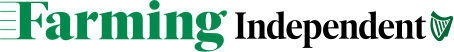# Useful formulae for working out nozzle selection, forward speed, application rate

Note: The symbol ‘/’ used in the formulae means divide, eg 6/2 = 3 means 6 divided by 2 = 3. Also, always work out the multiplication sections first and leave the division part of the formula to last.

? Nozzle output (litres/min) = Application rate (litres/ha) x forward speed (kph) x nozzle spacing (metres)/600.

? Forward speed (kph) = nozzle output (litres/min) x 600/application rate (l/ha) x nozzle spacing (metres).

? Application rate (l/ha) = nozzle output (l/min) x 600/ forward speed (kph) x nozzle spacing (metres).

(To convert from gallons per acre, multiply by 11.2 to give litres per hectare (l/ha)).

Calibration example

Say, if:

####Get the latest news from the Farming Independent team 3 times a week.

Nozzle output = 1.3 litres/min;

Forward speed = 7.8kph;

Nozzle spacing = 0.5m.

What would the application rate be?

? Application rate (litres/ha) = Nozzle output (litres/min) x 600/ forward speed (kph) x nozzle spacing (m)

= 1.3 x 600/ 7.8 x 0.5

= 780/ 3.9

= 200 litres/ha

Note: 600 is just a number and is always the same for this formula.

Forward speed can also be calculated or checked by using the formula below and driving at a fixed speed over a known distance between two points.

? Forward speed (kph) = 3.6 x distance measured (m) / time to travel distance (seconds)

For reference information used here and a lot more about spraying, visit www.teagasc.ie/publications/ti llage_crops2000/paper05.asp